International
Tables for
Crystallography
Volume A
Space-group symmetry
Edited by M. I. Aroyo

International Tables for Crystallography (2015). Vol. A, ch. 1.5, pp. 91-92

## Section 1.5.4.1.1. Determining the type of a symmetry operation

B. Souvignier,c G. Chapuisd and H. Wondratscheka

#### 1.5.4.1.1. Determining the type of a symmetry operation

| top | pdf |

In this section, a procedure for determining the types of symmetry operations and the corresponding symmetry elements is explained. It is a development of the method of geometrical interpretation discussed in Section 1.2.2.4. The procedure is based on the origin-shift transformations discussed in Sections 1.5.1and 1.5.2, and provides an efficient way of analysing the additional symmetry operations and symmetry elements. The key to the procedure is the decomposition of the translation partof a symmetry operationinto an intrinsic translation part, which is fixed by the linear partofand thus parallel to the geometric element of, and a location part, which is perpendicular to the intrinsic translation part. Note that the space fixed byand the space perpendicular to this fixed space are complementary, i.e. their dimensions add up to 3, therefore this decomposition is always possible.

As described in Section 1.2.2.4, the determination of the intrinsic translation part of a symmetry operationwith linear partof order k is based on the fact that the kth power ofmust be a pure translation, i.e.for some lattice translation. The intrinsic translation part ofis then defined as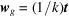.

The differenceis perpendicular toand it is called the location part of. This terminology is justified by the following observation: As explained in detail in Sections 1.5.1.3and 1.5.2.3, under an origin shift by, a columnof point coordinates is transformed to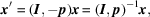making in particularthe new origin, and a matrix–column pairis transformed to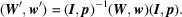Applied to the symmetry operation, known as the reduced symmetry operation in which the full translation part is replaced by the location part (thereby neglecting the intrinsic translation part), an origin shift byresults in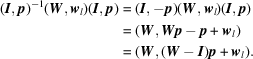This means that if it is possible to find an origin shift p such that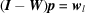, then with respect to the new origin the reduced symmetry operationis transformed to. But since the subspace perpendicular to the fixed space ofclearly does not contain any vector fixed by, the restriction of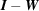to this subspace is an invertible linear transformation, and therefore for every location partthere is indeed a suitableperpendicular to the fixed space ofsuch that.

The fact that an origin shift bytransforms the translation part of the reduced symmetry operationtois equivalent tobeing a fixed point of, which can also be seen directly because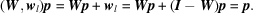Note that for one fixed pointof the reduced symmetry operation, the full set of fixed points, as defined in Section 1.2.4, is obtained by addingto the fixed vectors of, because for an arbitrary fixed point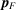ofone has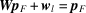and since also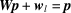one finds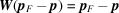, i.e. the difference between two fixed points is a vector that is fixed by. In other words, the geometric element ofis the space fixed by, translated such that it runs through.

Finally, in order to determine the symmetry element of the symmetry operation correctly, it may be necessary to reduce the intrinsic translation partby a lattice translation in the fixed space of.

Summarizing, the types of symmetry operationsand their symmetry elements can be identified as follows:

 (i) Decompose the translation partas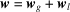, whereandare mutually perpendicular and the intrinsic translation partis fixed by the linear partof. (ii) Determine a shift of originsuch that, i.e. such thatis a fixed point of the reduced operation. (iii) For the correct determination of the defining operation of the symmetry element it may be necessary to reduce the intrinsic translation partby a lattice translation in the fixed space of, thus yielding a coplanar or coaxial equivalent symmetry operation.

This analysis allows one to read off the types of the symmetry operations and of the corresponding symmetry elements that occur for the coset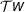of. The following two sections provide examples illustrating that in some cases the coset does not contain symmetry operations belonging to symmetry elements of different type, while in others it does.